# AP Statistics : How to do two-sided tests of significance

## Example Questions

### Example Question #1 : How To Do Two Sided Tests Of Significance

Bob and Alvin suspect that the weight of the average man in Jackson, Mississippi is significantly different than the weight of the average man in Green Bay, Wisconsin. They sample 100 men in Jackson and find that the average weight is 191 lbs, and the sample standard deviation is 30 lbs.

They sample 100 men in Green Bay and find that the average weight is 184, and the sample standard deviation is 25.

Bob says that they should present a 95% 1-tailed test where the alternate hypothesis is: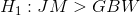and the null hypothesis is: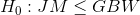Alvin disagrees; he says that a one-tailed test assumes that we already suspected a higher weight in Jackson. He recommends a 95% 2-tailed test where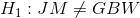and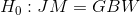.

Show why the 1-tailed test rejects its null hypothesis and the 2-tailed test fails to reject its null hypothesis. Provide the following:

1) The Z-value for a 1-tailed 95% test.

2) The Z-value for a 2-tailed 95% test.

3) The Z-value for the sample difference of 7.

1) 1.645

2) 1.96

3) 1.81

1) 1.645

2) 1.96

3) 1.67

1) 1.645

2) 1.96

3) 1.79

1) 1.645

2) 1.96

3) 1.72

1) 1.645

2) 1.96

3) 1.91

1) 1.645

2) 1.96

3) 1.79

Explanation:

The variance for the sample difference is: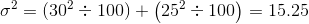The standard deviation for the sample difference is: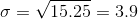The Z-value for a difference of 7 is: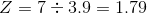The sample difference Z-value is greater than the 1-tailed Z-value (causing us to reject the null hypothesis) and is less than the 2-tailed Z-value (causing us to fail to reject the null hypothesis).